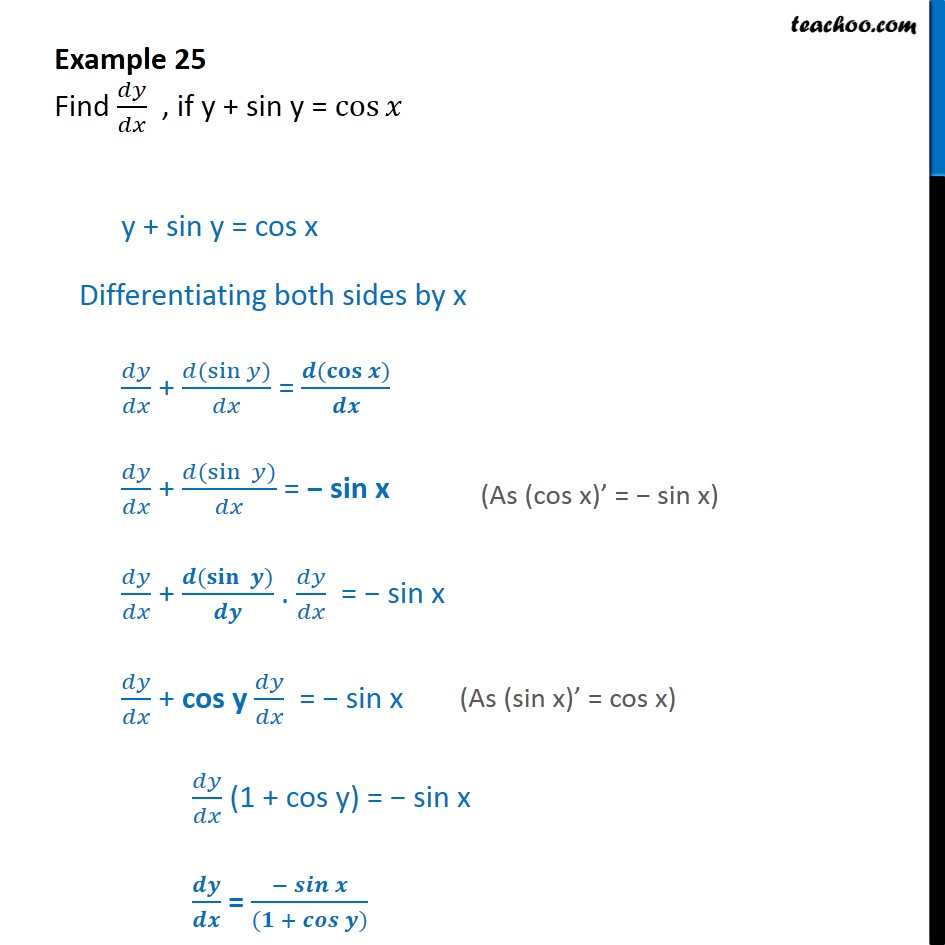1. Chapter 5 Class 12 Continuity and Differentiability
2. Concept wise
3. Finding derivative of Implicit functions

Transcript

Example 25 Find / , if y + sin y = cos y + sin y = cos x Differentiating both sides by x / + ( (sin ) )/ = ( ( ) )/ / + ( ( sin ) )/ = sin x / + ( ( ) )/ . / = sin x / + cos y / = sin x / (1 + cos y) = sin x / = ( )/(( + ) )

Finding derivative of Implicit functions